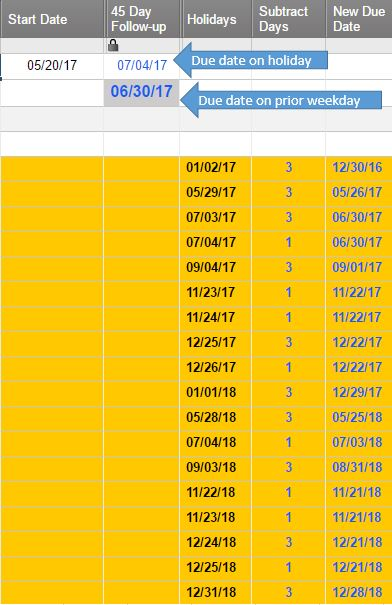#### Welcome to the Smartsheet Forum Archives

The posts in this forum are no longer monitored for accuracy and their content may no longer be current. If there's a discussion here that interests you and you'd like to find (or create) a more current version, please Visit the Current Forums.

# Due date PRIOR to weekend or holiday

I have a one date upon which all other due dates are calculated.

We have holidays set up for the account.

I need the due dates to fall on the weekday PRIOR to the due date if it is a weekend or holiday.  It appears the Workday and Networkday functions fall on the following week day, which doesn't work for me.

This formula calculates if the date falls on a weekend.  If not a weekend, 45 days, if a Sunday 43 days, if a Saturday 44 days.  How would I add in Holidays?

=IF(WEEKDAY([Start Date]17 + 45) = 1, [Start Date]17 + 43, IF(WEEKDAY([Start Date]17 + 45) = 7, [Start Date]17 + 44, [Start Date]17 + 45))

If the start date is 5/20/17, adding 45 days causes it to fall on 7/4/17.  Since 7/3 and 7/4 are company holidays, I need the 45 day due date to be 6/30/17, the previous workday.

Thank you.

Tags:

• Hello,

You can use the WORKDAY function for this: https://help.smartsheet.com/function/workday

You'd need to add the holiday dates to your sheet, possibly in a hidden column.

• Thank you for your response, however, that doesn't work.  It's not 45 workdays, it's 45 days, however, if the 45th day lands on a weekend or holiday, the due date is the business day prior.

This is how I do it in Excel where \$B\$5 is the start date and the word "holidays" in the formula is a named range of the holiday dates:

=IF(\$B\$5 = "", "", \$B\$5+45-SMALL(IF(WEEKDAY(\$B\$5+45-{0,1,2,3,4,5},2)<6,IF(COUNTIF(holidays,\$B\$5+45-{0,1,2,3,4,5})=0,{0,1,2,3,4,5})),1))

• I think I've got this figured out.

Screenshot shows the layout, below are the formulas that force a 45 day due date to fall on the weekday prior taking weekends and holidays into account.  (It's 45 days, not 45 workdays.)

Holidays start on row 5 and are always on a weekday

Weekday 1 = Sunday

Subtract Days formula:

=IF(WEEKDAY(Holidays5) = 2, 3, IF(WEEKDAY(Holidays5) > 2, 1))

New Due Date formula: (takes into account two holidays next to each other)

=IF(ISERROR(INDEX(Holidays\$5:[Subtract Days]\$22, MATCH(Holidays5 - [Subtract Days]5, Holidays\$5:Holidays\$22, 0), 2)), Holidays5 - [Subtract Days]5, ((Holidays5 - [Subtract Days]5) - INDEX(Holidays\$5:[Subtract Days]\$22, MATCH(Holidays5 - [Subtract Days]5, Holidays\$5:Holidays\$22, 0), 2)))

45 Day formula removing weekends & holidays

=IF(ISERROR(INDEX(\$Holidays\$5:\$[New Due Date]\$22, MATCH(IF(WEEKDAY(\$[Start Date]\$1 + 45) = 1, \$[Start Date]\$1 + 43, IF(WEEKDAY(\$[Start Date]\$1 + 45) = 7, \$[Start Date]\$1 + 44, \$[Start Date]\$1 + 45)), \$Holidays\$5:\$Holidays\$22, 0), 3)), IF(WEEKDAY(\$[Start Date]\$1 + 45) = 1, \$[Start Date]\$1 + 43, IF(WEEKDAY(\$[Start Date]\$1 + 45) = 7, \$[Start Date]\$1 + 44, \$[Start Date]\$1 + 45)), INDEX(\$Holidays\$5:\$[New Due Date]\$22, MATCH(IF(WEEKDAY(\$[Start Date]\$1 + 45) = 1, \$[Start Date]\$1 + 43, IF(WEEKDAY(\$[Start Date]\$1 + 45) = 7, \$[Start Date]\$1 + 44, \$[Start Date]\$1 + 45)), \$Holidays\$5:\$Holidays\$22, 0), 3))This discussion has been closed.